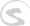Documents
Writing Docs Gigedit SFZ Instrument Scripts NKSP Language NKSP Reference

# sh_right()

Calculates right bit shifted values. The original value of the 1st argument is bit shifted to the right as many times as requested by the 2nd argument and the result of this bit shift operation is returned by this function.

### Function Prototype

`sh_right(number, shifts) `

### Arguments

Argument Name Data Type Description
`number` Integer Number Original input value to be shifted.
[required]
`shifts` Integer Number Amount of times the original value should be shifted to the right.
[required]

### Return Value

Data Type Description
Integer Number Bit shifted result.

### Remarks

This functions accepts finalness for its argument `number`. The return value's finalness will be identical to `number`'s one.

### Examples

`sh_left()`, `msb()`, `lsb()`